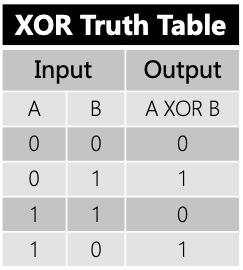# XOR 异或运算在计算机中的应用### 1. 什么是异或运算

xor是一个数学运算符。它应用于逻辑运算。异或的数学符号为“⊕”，计算机符号为“xor”。如果a、b两个值不相同，则异或结果为1。如果a、b两个值相同，异或结果为0。

﻿

﻿﻿

### 2. 异或运算的特性

• 交换律：A⊕B = B⊕A

• 结合律：A⊕(B⊕C) = (A⊕B)⊕C

• 恒等律：X⊕0 = X

• 归零律：X⊕X = 0

• 自反律：A⊕B⊕B = A⊕(B⊕B) = A⊕0 = A

### 3. XOR在计算机中的应用

##### 3.1 快速比较两值是否想等
r = a xor bif r == 0     print(“a == b”)else    print(“a != b")
##### 3.2 检验一个数字中1的个数的奇偶（奇偶校验）

1 ^ 0 ^ 1 ^ 0 ^ 0 ^ 0 ^ 0 ^ 1 = 1

##### 3.3 不使用中间变量，交换两个变量的值
a = a ^ b;b = a ^ b; //a ^ b ^ b = a ^ 0 = a;a = a ^ b;

a^a^b^b^c^c^d

= 0^0^0^d

= 0^d

= d

##### 3.5 密码学中对称加密解密

message XOR key // cipherText

cipherText XOR key // message

﻿## 评论